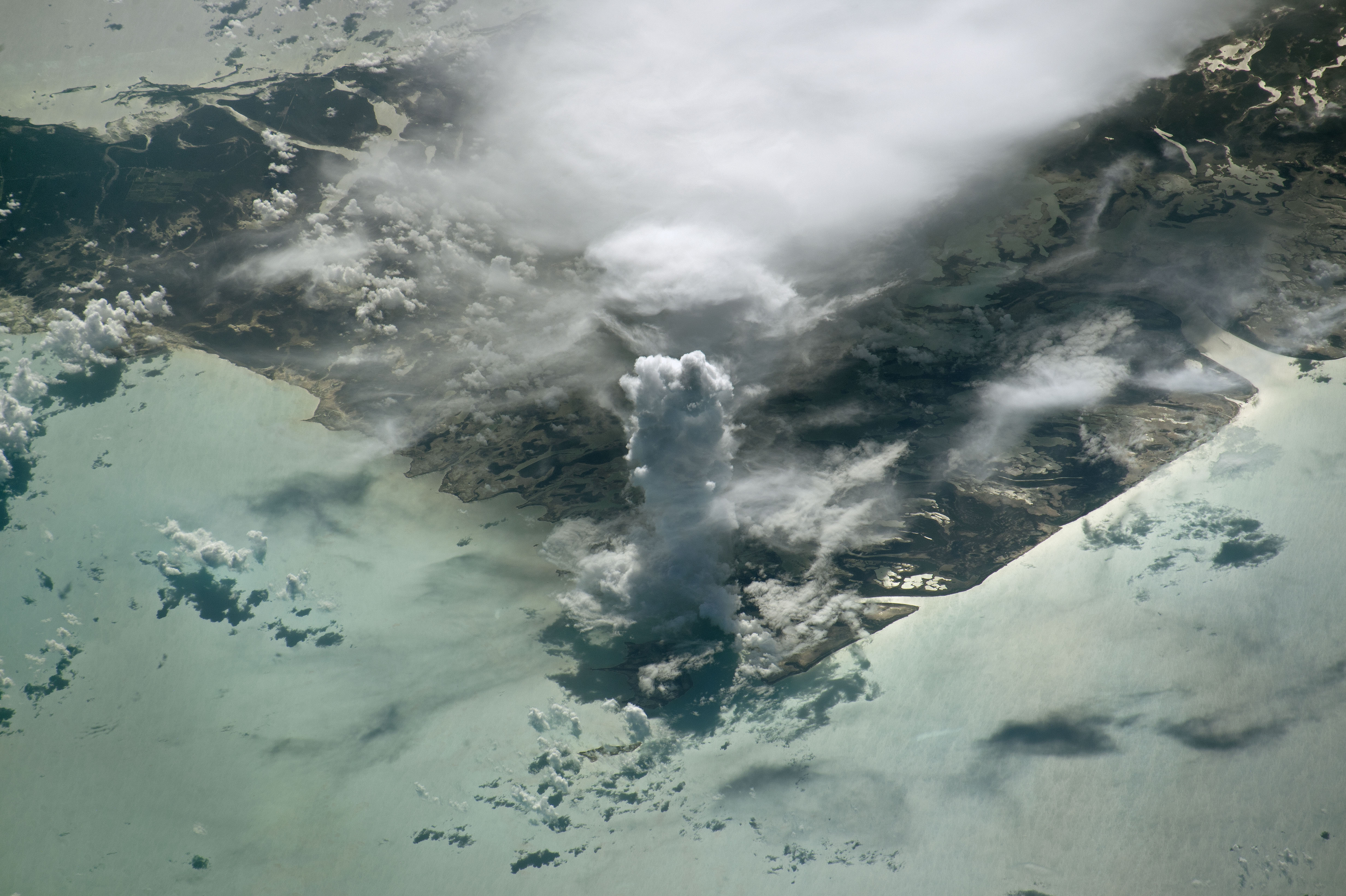# Modeling atmospheric turbulence and convectionThe landform in the photograph shown above is Andros Island, in the Bahamas. Andros Island is about 60 km wide, close to the horizontal resolution that current climate models can afford. Climate models leverage our knowledge of the equations of fluid motion to forecast future changes in the atmosphere and the ocean due to, among other things, anthropogenic forcing.

Although all climate models robustly forecast global warming as a consequence of rising $CO_2$ levels, predictions for just how fast the Earth will warm in the future vary. The largest contribution to this uncertainty comes from clouds. Clouds have an outsized effect on climate due to their radiative properties. However, the phenomena responsible for the formation of clouds act on scales smaller than the resolution of climate models, as seen in the picture of Andros Island, and thus cannot be resolved explicitly. The myriad methods used by models to compensate for this fact leads to a spread in model predictions.

Mathematically, the effect of finite resolution on climate model forecasts can be expressed in a compact way if we consider the dynamics of an atmospheric tracer, such as the specific humidity $q$,

$\dfrac{\partial (\rho q)}{\partial t} = - \nabla\cdot (\rho \mathbf{u} q) + \rho S_{q},$

where $\rho$ is the air density, $S_{q}$ represents all sources and sinks of specific humidity and $\mathbf{u}$ is the velocity vector. If we average this equation over a climate model grid box, denoting averages as $(\bar{\cdot})$ and deviations from the average as $(\cdot’)$,

$\dfrac{\partial (\bar{\rho} \bar{q})}{\partial t} = - \nabla\cdot (\bar{\rho} \bar{\mathbf{u}} \bar{q}) - \nabla\cdot (\bar{\rho} \overline{\mathbf{u}'q'}) + \bar{\rho} \bar{S_{q}},$

a new term arises, $\nabla\cdot (\rho \overline{\mathbf{u}’q’})$. This flux divergence represents the effect that the small-scale correlations between the wind speed and moisture have on the grid average $\bar{q}$. Climate models can only compute grid-mean quantities (i.e., $\bar{q}$ or $\bar{\mathbf{u}}$), which means that this flux divergence encapsulates climate model errors due to a lack of resolution.

Consider again the picture of Andros Island above. Towering castellanus clouds like the one at the forefront of the scene occur when moist ($q’ > 0$) warm air rises at high vertical speed ($\mathbf{u}’\cdot \mathbf{k} > 0$) due to convection. If instead, rising air parcels were forecast to be drier ($q \approx \bar{q}$) and roughly quiescent ($\mathbf{u}\cdot \mathbf{k} \approx 0$), many of the clouds in the scene would disappear.

Climate models can reduce errors associated with this unknown flux divergence through the use of parameterizations $f(\cdot)$ based on the variables they do have access to, such that ideally they have the same aggregate effect on the grid mean variables,

$\nabla\cdot (\bar{\rho} \overline{\mathbf{u}'q'}) \approx \nabla\cdot (f(\bar{\mathbf{u}}, \bar{q}, \dots)).$

Improving such parameterizations is an active research topic that has the potential to reduce biases and uncertainty in climate projections. These parameterizations must include the effects of all unresolved dynamical processes, and ideally represent the interaction between subgrid processes consistently.

Two unresolved processes of great dynamic importance are turbulence and convection. A particularly powerful framework to parameterize both processes in a consistent way is the one provided by Eddy-Diffusivity Mass-Flux (EDMF) schemes. In this framework, the problem of finding an optimal parameterization $f(\cdot)$ representing the complex unresolved dynamics in the lower troposphere can be broken down into three fundamental problems:

• What drives the mass exchange between updrafts, like the towering cloud in the picture, and the surrounding environment?
• What controls the vertical turbulent mixing of air parcels?
• How strong is the environmental resistance to the ascent of updrafts?

In a series of articles (Cohen et al. 2020, Lopez-Gomez et al. 2020, He et al. 2021), we propose solutions to these three questions, in the context of an EDMF scheme of turbulence and convection. By implementing our proposed parameterization in a single column of a climate model, we show that it is able to correctly represent both low and deep clouds found near the tropics, as well the vertical structure of the atmosphere in regions as far from Andros Island as the Bering Sea.